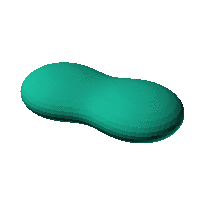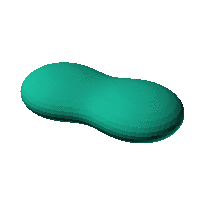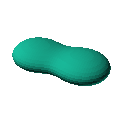Blob Farm# blob24The blob in all its glory:Peanut shape

 Click on the snapshot to download the blob's stl file.Octave Code:
```  # name of the blob
project = "blob24";

function w = f(x2,y2,z2,c,r,e)
x  = (x2-c(1))/r(1);
y  = (y2-c(2))/r(2);
z  = (z2-c(3))/r(3);
# function at origin must be <0, and >0 far enough away.  w=0 defines the surface
w=(-34.93)+(-20.5).*x.^2+x.^4+4.5.*y.^2+2*x.^2.* ...
y.^2+y.^4+18.*z.^2+8.*x.^2.*z.^2+8.*y.^2.*z.^2+ ...
16.*z.^4;;

endfunction;

step = 4;  # grid pitch in mm  start with 4mm to see the shape quickly.  Once you have it just right, change to 2mm for printing

c_outer = [0,0,0];
r_outer = [20,20,20];

source("../octave/func2stl_v01.m");  # do all the calculations
```
GNU Octave